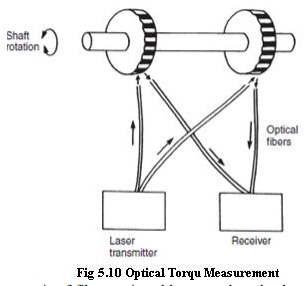Home | | Metrology and Measurements | Torque Measurement

# Torque Measurement

Measurement of applied torques is of fundamental importance in all rotating bodies to ensure that the design of the rotating element is adequate to prevent failure under shear stresses.

TORQUE MEASUREMENT

·        Measurement of applied torques is of fundamental importance in all rotating bodies to ensure that the design of the rotating element is adequate to prevent failure under shear stresses.

·        Torque measurement is also a necessary part of measuring the power transmitted by rotating shafts.

·        The four methods of measuring torque consist of

·        Measuring the strain produced in a rotating body due to an applied torque

·        An optical method

·        Measuring the reaction force in cradled shaft bearings

·        Using equipment known as the Prony brake.

Measurement of Induced Strain

Measuring the strain induced in a shaft due to an applied torque has been the most common method used for torque measurement in recent years. The method involves bonding four strain gauges onto a shaft as shown in Figure, where the strain gauges are arranged in a d.c. bridge circuit. The output from the bridge circuit is a function of the strain in the shaft and hence of the torque applied. It is very important that positioning of the strain gauges on the shaft is precise, and the difficulty in achieving this makes the instrument relatively expensive. This technique is ideal for measuring the stalled torque in a shaft before rotation commences. However, a problem is encountered in the case of rotating shafts because a suitable method then has to be found for making the electrical connections to the strain gauges. One solution to this problem found in many commercial instruments is to use a system of slip rings and brushes for this, although this increases the cost of the instrument still further.Optical Torque Measurement

Optical techniques for torque measurement have become available recently with the development of laser diodes and fiber-optic light transmission systems. One such system is shown in Figure. Two black-and-white striped wheels are mounted at either end of the rotating shaft and are in alignment when no torque is applied to the shaft. Light from a laser diode light source is directed by a pair of fiber-optic cables onto the wheels. The rotation of the wheels causes pulses of reflected light, which are transmitted back to a receiver by a second pair of fiber-optic cables. Under zero torque conditions, the two pulse trains of reflected light are in phase with each other. If torque is now applied to the shaft, the reflected light is modulated. Measurement by the receiver of the phase difference between the reflected pulse trains therefore allows the magnitude of torque in the shaft to be calculated. The cost of such instruments is relatively low, and an additional advantage in many applications is their small physical size.Reaction Forces in Shaft Bearings

Any system involving torque transmission through a shaft contains both a power source and a power absorber where the power is dissipated. The magnitude of the transmitted torque can be measured by cradling either the power source or the power absorber end of the shaft in bearings, and then measuring the reaction force, F, and the arm length, L, as shown in Figure. The torque is then calculated as the simple product, FL. Pendulum scales are used very commonly for measuring the reaction force. Inherent errors in the method are bearing friction and windage torques. This technique is no longer in common use.Prony Brake

The  Prony  brake  is  another  torque- measuring system that is now uncommon. It is used to measure the torque in a rotating shaft and consists  of  a  rope  wound  round  the  shaft,  as illustrated  in  Figure.  One  end  of  the  rope  is attached to a spring balance and the other end carries a load in the form of a standard mass, m.

If the measured force in the spring balance is Fs, then the effective force, Fe, exerted by the rope on the shaft is given by

Fe = mg - Fs

If the radius of the shaft is Rs and that of the rope is Rr, then the effective radius, Re, of the rope and drum with respect to the axis of rotation of the shaft is given by

Re = Rs + Rr

The torque in the shaft, T, can then be calculated as

T= FeRe

While this is a well-known method of measuring shaft torque, a lot of heat is generated because of friction between the rope and shaft, and water cooling is usually necessary.Study Material, Lecturing Notes, Assignment, Reference, Wiki description explanation, brief detail
Mechanical : Metrology and Measurements : Measurement of Power, Flow and Temperature Related Properties : Torque Measurement |